# Discrete data

Here you will learn about discrete data, including what it is, how to collect it and how to graph and analyze it.

Students first learn to work with discrete data in first grade and expand their knowledge and use of data as they progress through the grades.

## What is discrete data?

Discrete data includes numeric data values that are countable.

Some common examples of discrete data sets include:

• The shoe size of everyone in a family
• Number of likes on a post over time
• Number of students in each classroom of a school
• Number of adoptions at a pet shelter each month

Discrete data can be collected through questionnaires, surveys, interviews, or observations.

Once the data has been collected, it can be graphed, which provides a visualization of the data. Pictographs and bar graphs (or bar charts) are common ways to represent discrete data. You can also use histograms, box plots, pie charts, or frequency tables, however note that these can also be used for continuous data.

Besides graphs, data analysis of discrete data may include calculating the mean, median, range or mode. Particularly if the data is being used for statistical analysis.

Finally, the graphs and/or measures of center can be used to answer questions about the data.

For example,

How many cats do the students in my class have?

The data is discrete, because there are a limited number of answers. You can’t have 0.5 of a cat, just like you can’t have 10,000.

Once the data is collected, you can use a bar graph to display it.

Now, you can use the data in the table and the bar graph to answer questions like…

• What is the most common number of cats? 0 cats
• What is the most number of cats? 5 cats
• How many more students have 2 cats than 1 cat? 2 students

### What is discrete data?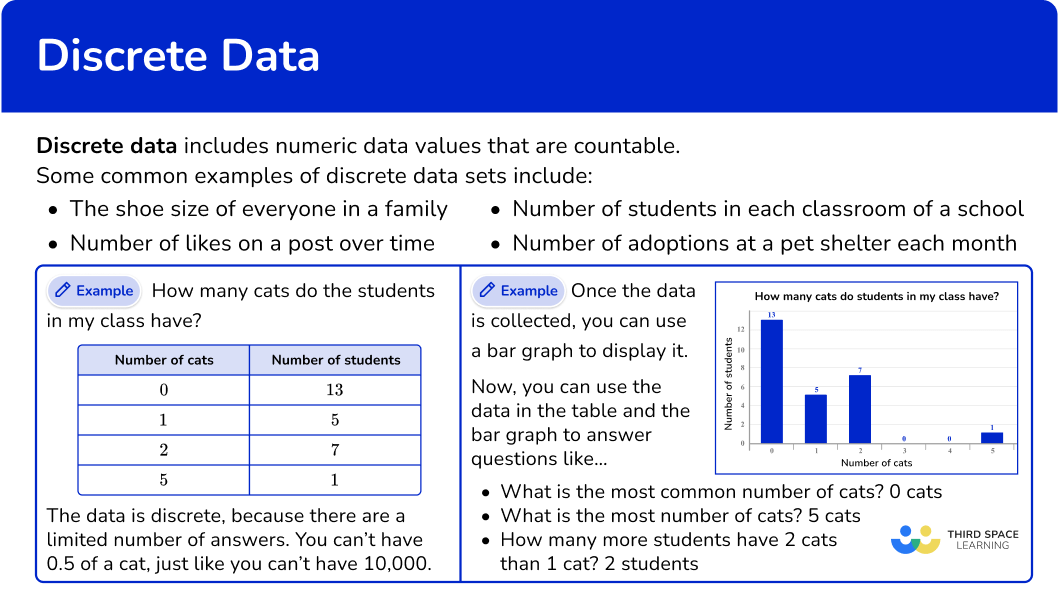## Common Core State Standards

• Grade 1 – Measurement and Data (1.MD.C.4)
Organize, represent, and interpret data with up to three categories; ask and answer questions about the total number of data points, how many in each category, and how many more or less are in one category than in another.

• Grade 2 – Measurement and Data (2.MD.D.9)
Generate measurement data by measuring lengths of several objects to the nearest whole unit, or by making repeated measurements of the same object. Show the measurements by making a line plot, where the horizontal scale is marked off in whole-number units.

• Grade 3 – Measurement and Data (3.MD.B.3)
Draw a scaled picture graph and a scaled bar graph to represent a data set with several categories. Solve one- and two-step “how many more” and “how many less” problems using information presented in scaled bar graphs.

For example, draw a bar graph in which each square in the bar graph might represent 5 pets. Generate measurement data by measuring lengths using rulers marked with halves and fourths of an inch.

Show the data by making a line plot, where the horizontal scale is marked off in appropriate units—whole numbers, halves, or quarters.

• Grade 4 – Measurement and Data (4.MD.B.4)
Make a line plot to display a data set of measurements in fractions of a unit (\cfrac{1}{2}, \, \cfrac{1}{4}, \, \cfrac{1}{8}) . Solve problems involving addition and subtraction of fractions by using information presented in line plots.

For example, from a line plot find and interpret the difference in length between the longest and shortest specimens in an insect collection.

• Grade 5 – Measurement and Data (5.MD.B.2)
Make a line plot to display a data set of measurements in fractions of a unit (\cfrac{1}{2}, \, \cfrac{1}{4}, \, \cfrac{1}{8}) . Use operations on fractions for this grade to solve problems involving information presented in line plots.

For example, given different measurements of liquid in identical beakers, find the amount of liquid each beaker would contain if the total amount in all the beakers were redistributed equally.

• Grade 6 – Statistics and Probability (6.SP.B.5)
Summarize numerical data sets in relation to their context.

## How to recognize discrete data

In order to recognize discrete data:

1. Look for data that uses only numbers.
2. Look for numbers that are a finite set.

## Discrete data examples

### Example 1: recognizing types of data

Is the data set below discrete? Why or why not?

1. Look for data that uses only numbers.

The data shows how many cans were donated by how many children. This data uses only numbers.

2Look for numbers that are a finite set.

The number of cans donated is NOT continuous. You can’t donate \cfrac{1}{3} of a can and at some point a number like 10,000,000 is unreasonable. The data has a set number of possible responses, so it is discrete.

### Example 2: recognizing types of data

Is the data set below discrete? Why or why not?

Look for data that uses only numbers.

Look for numbers that are a finite set.

### Example 3: recognizing types of data

Is the data set below discrete? Why or why not?

Look for data that uses only numbers.

## How to analyze discrete data

In order to analyze discrete data:

1. Collect discrete data.
2. Choose a graph or measure of center to calculate.
3. Answer questions based on the data analysis.

### Example 4: analyzing and then answering a question – discrete data

Scenario: Students in your class use leaves as a part of an art project. You wonder, “How many leaves did students in my class use for the art project?”

Collect discrete data.

Choose a graph or measure of center to calculate.

Answer questions based on the data analysis.

### Example 5: analyzing and then answering a question – discrete data

Scenario: A grocery store keeps track of how many customers visit each day.

Collect discrete data.

Choose a graph or measure of center to calculate.

Answer questions based on the data analysis.

### Example 6: analyzing and then answering a question – discrete data

Scenario: You wonder, “What year were my family members older than me born?”

Collect discrete data.

Choose a graph or measure of center to calculate.

Answer questions based on the data analysis.

### Teaching tips for discrete data

• Include activities where students collect their own discrete data by creating surveys, conducting interviews or doing experiments. Then analyzing what they’ve collected and drawing conclusions based on the data. This will help students develop a complete understanding of discrete data.

• Give students a mixture of categorical, discrete and continuous data and have them sort each set into the correct category. Then have students discuss similarities and differences between the data types.

### Easy mistakes to make

• Confusing discrete vs. continuous data
Since both involve numbers, it is not always easy for students to understand the difference of discrete data vs. continuous data.

Use a number line to explain the key difference – highlighting that discrete data is data that only includes the specific values (like the ones shown on the number line), but the continuous data includes all values (including the ones between the numbers shown – an infinite number of possible values).

Examples of discrete data (specific values on the number line – only those labeled)…

Examples of continuous data (all values on the number line – those labeled and not labeled)…

• Thinking discrete data is only whole numbers
While discrete data sets often include whole numbers, this is not the only type of numbers that can be used.

For example,
Shoe sizes are a discrete data set and include sizes like 6.5 and 9.5, which include decimals.

### Practice discrete data questions

1. Which question will collect discrete data?What is the temperature outside?What day of the week is it?Discrete data collects only numbers.

These questions have answers that are NOT numbers:

• What day of the week is it? Possible answer: Monday, Tuesday…

Discrete data has a set number of possible responses.

This question does NOT have a set number of responses:

• What is the temperature outside? 5^{\circ}, 5.1^{\circ}, 5.13^{\circ},   5.137^{\circ},   etc. – the possible temperature answers are infinite

The question “What is your shoe size?” is answered with a finite set of numbers, so it will provide a discrete data set.

2. Which question will collect discrete data?

What month were you born?How many cars in the parking lot are blue?What speed does a turtle move?Discrete data collects only numbers.

These questions have answers that are NOT numbers:

• What month were you born? Possible answers: March, April, May…

Discrete data has a set number of possible responses.

This question does NOT have a set number of responses:

• What speed does a turtle move? 4 \; mph, \, 3.9 \; mph, \, 3.94 \; mph, \, 3.9453 \; mph etc. – the possible speed answers are infinite.

The question “How many cars in the parking lot are blue?” is answered with a finite set of numbers, so it will provide a discrete data set.

3. Which question will NOT collect discrete data?

How many seconds does it take to run 100 m?How many siblings do you have?How many tickets were sold each day?How many students are in each class?Discrete data uses only numbers and all the questions have numbers as answers.

Discrete data has a set number of possible responses.

This question does NOT have a set number of responses:

• How many seconds does it take to run 100 \; m? \, 11 seconds, 11.1 seconds, 11.13 seconds, 11.137 seconds, etc. – the possible amount of time answers are infinite.

4. Which table shows discrete data?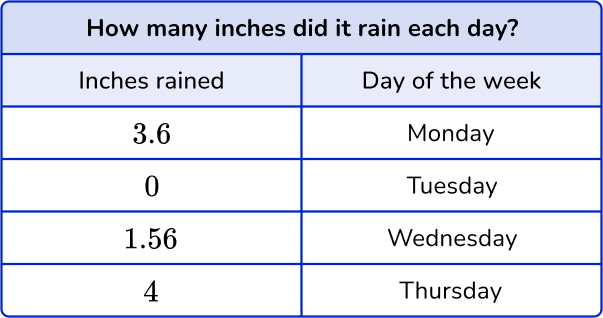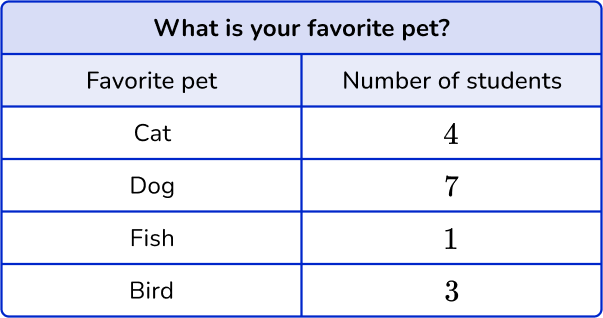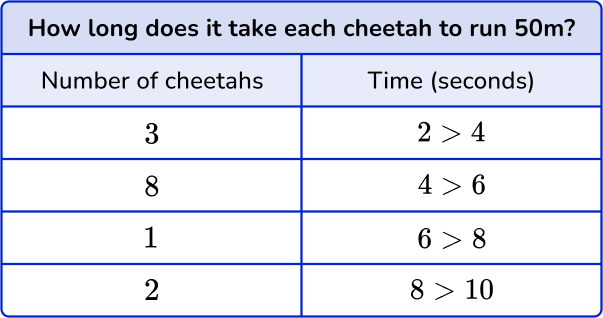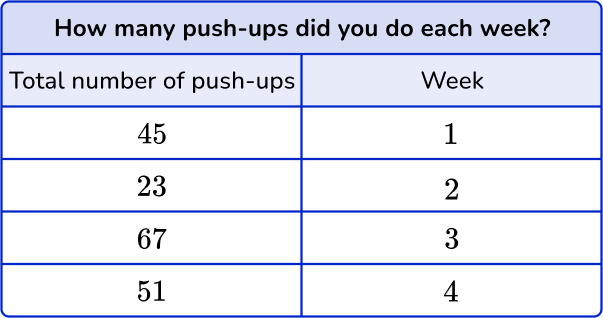Discrete data collects only numbers.

The second table has answers that are NOT numbers:

• What is your favorite pet? Possible answers: cat, dog, fish, bird

Discrete data has a set number of possible responses.

The first and third table do NOT have a set number of responses:

• How many inches did it rain each day? 1.2 inches, 1.23 inches, 1.231 inches etc. – the possible length answers are infinite.

• How long does it take each cheetah to run 50 \; m? \; 2 seconds, 2.4 seconds, 2.43 seconds, etc. – the possible amount of time answers are infinite.

The last table includes data for the question “How many push-ups did you do each week?” which is answered with a finite set of numbers, so the data set includes only discrete values.

5. Which graph does NOT show discrete data?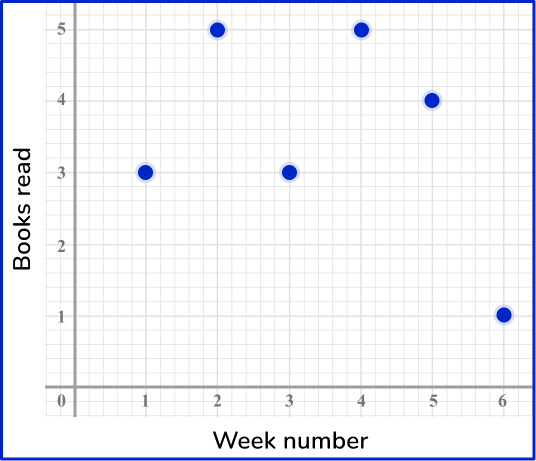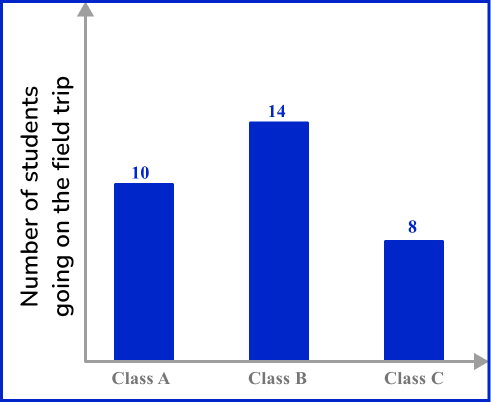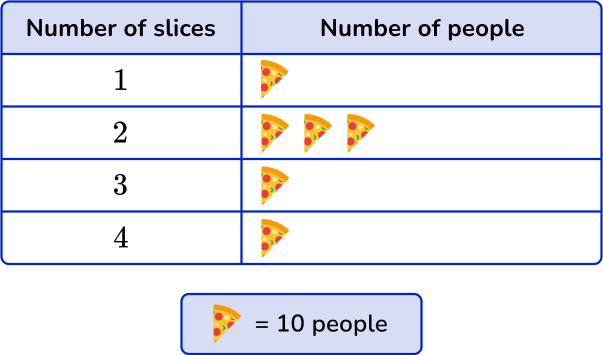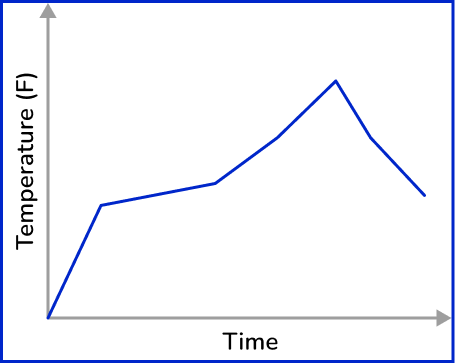Discrete data collects only numbers – all graphs show a numerical data set.

Discrete data has a set number of possible responses.

The last graph does NOT have a set number of responses:

• What is the temperature throughout the day? 50 degrees, 50.1 degrees, 50.13 degrees, 50.132 degrees, etc. – the possible answers are infinite.

A line graph always represents continuous data.

6.How many more people ate exactly 2 slices than 1 slice?

210201Each pizza represents 10 people.

So 10 people had 1 slice and 30 people had exactly 2 slices.

30 slices – 10 slices = 20 slices

## Discrete data FAQs

Are integers continuous?

The set of integers is an infinite set. However, data wise they are considered a countable set and therefore used to represent discrete data.

Is ordinal data discrete?

No, though ordinal data is represented with digits, they are used to define an order, not an amount. Knowing that one runner finished 1 st and another 2 nd, tells you the order in which they finished, but gives no indication of the amount of time it took each runner or the numerical value of the distance between their finishes.

## Still stuck?

At Third Space Learning, we specialize in helping teachers and school leaders to provide personalized math support for more of their students through high-quality, online one-on-one math tutoring delivered by subject experts.

Each week, our tutors support thousands of students who are at risk of not meeting their grade-level expectations, and help accelerate their progress and boost their confidence.